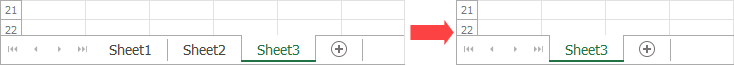.NET Framework 4.5.2+

# WorksheetCollection Interface

A collection of worksheets in a workbook.

## Declaration

``````public interface WorksheetCollection :
ISimpleCollection<Worksheet>,
IEnumerable<Worksheet>,
IEnumerable,
ICollection``````

## Remarks

Use the WorksheetCollection object’s members to access, add, and remove worksheets.

### Access a Worksheet

Use the WorksheetCollection.Item property to access a worksheet in a workbook.

#### Obtain the Worksheet at the Specified Index

``````using DevExpress.Spreadsheet;
// ...

// Access the worksheet collection.
WorksheetCollection worksheets = workbook.Worksheets;

// Access the first worksheet.
Worksheet worksheet1 = workbook.Worksheets;
``````

A worksheet index is zero-based. It specifies the worksheet position within a collection.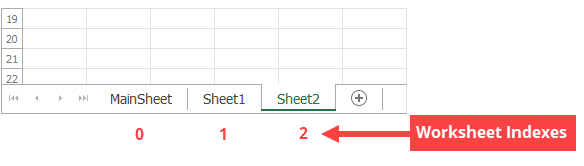#### Obtain the Worksheet with a Given Name

``````using DevExpress.Spreadsheet;
// ...

//  Access the worksheet collection.
WorksheetCollection worksheets = workbook.Worksheets;

// Access the worksheet with the specified name.
Worksheet worksheet2 = workbook.Worksheets["MainSheet"];
``````

A worksheet name is unique within the collection and is shown on a worksheet tab.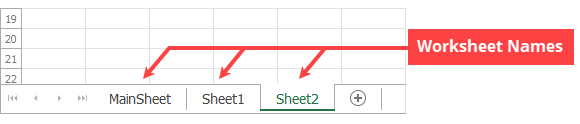### Set an Active Worksheet

Use the WorksheetCollection.ActiveWorksheet property to specify the active worksheet in a workbook.

``````using DevExpress.Spreadsheet;
// ...

// Set "Sheet2" as the active worksheet.
workbook.Worksheets.ActiveWorksheet = workbook.Worksheets["Sheet2"];
``````

Use the WorksheetCollection.Add method to add a worksheet to the end of the worksheet collection.

``````using DevExpress.Spreadsheet;
// ...

// Add a worksheet with the default name.
// Default names are "Sheet1", "Sheet2", ..., "SheetN".

// Add new worksheets with the specified names.

``````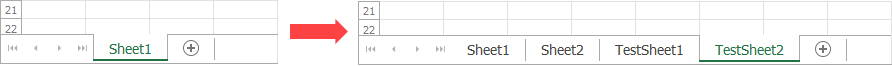The WorksheetCollection.Insert method allows you to insert a worksheet at the specified position in the collection.

``````using DevExpress.Spreadsheet;
// ...

// Insert a worksheet at the second position in the workbook.
workbook.Worksheets.Insert(1, "TestSheet3");

// Insert a worksheet with the default name at the fourth position in the workbook.
workbook.Worksheets.Insert(3);
``````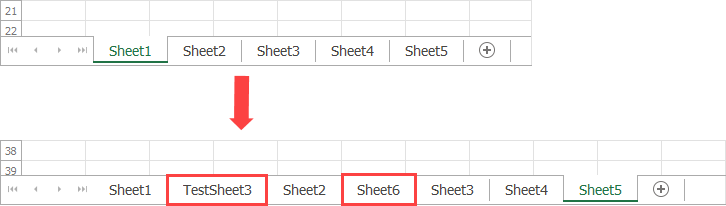### Delete a Worksheet

Use the following methods to delete a worksheet:

Note

A workbook must contain at least one visible worksheet.

``````using DevExpress.Spreadsheet;
// ...

// Delete the first worksheet from the workbook.
workbook.Worksheets.RemoveAt(0);

// Delete the "Sheet2" worksheet from the workbook.
workbook.Worksheets.Remove(workbook.Worksheets["Sheet2"]);
``````